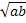A | B | C | D | E | F | G | H | I | J | K | L | M | N | O | P | Q | R | S | T | U | V | W | X | Y | Z

# Mathematics-Equation Corner

Laws of Indices::-
(1)
am x an = am + n

(2) am / an = a
m - n

(3) (am)n = a
mn

(4) (ab)n = anb
n

(5) (a / b)n = an / bn

(6) a0 = 1

Laws of Surds ::-

(1)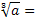a1/3

(2)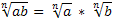(3)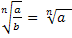/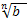Sum of n Terms ::-

(1 + 2 + 3 +  . . . . + n ) = n ( n + 1 ) / 2

Sum of squares n Terms ::-

( 12 + 22 + . . . . . .+ n2 ) = n (n + 1 ) (2n + 1) / 6

Sum of cube n Terms ::-

( 13 +  23 + 33 + . . . . .+ n3 ) = n2 ( n + 1 )2 / 4

Arithmetic Progression ( A.P ) :: -

a, a + d, a + 2d, . . .  are said to be in A.P,  in which a = first term, d = common difference

Then nth term of A.P  =   a + ( n - 1 ) d

Sum of n terms = n [ 2a + ( n - 1 ) d ]/ 2

Geometric Progression ( G.P ) ::-

a, ar2, ar3, ar4 , . . . . . are said to be G.P, in which a = first term and r = common ratio.

So nth term = a ( 1 - rn ) / ( 1 - r ) if r < 1
= a ( rn - 1 ) / ( r - 1 ) if r > 1

H.C.F :: -

H. C. F of Numerators / L.C.M of Denominators

L.C.M ::-

L.C.M of Numerators / H.C.F of Denominators

Basic Formulae :: -

( 1 ) ( a + b ) ( a - b ) =  ( a2 - b2 )

( 2 ) ( a + b )2 = ( a2 + b2 + 2ab )

( 3 ) ( a - b )2 = ( a2 + b2 - 2ab )

( 4 ) ( a + b + c )2 = (  a2 + b2 + c2 + 2ab + 2bc + 2ac )

( 5 ) ( a3 + b3 ) = ( a + b ) ( a2 - ab + b2 )

( 6 ) ( a3 - b3 ) = ( a - b ) ( a2 + ab + b2 )

( 7 ) ( a3 + b3 + c3  - 3abc ) = ( a + b + c ) ( a2 + b2 + c2 - ab - bc - ac )

( 8 ) If ( a + b + c ) = 0, then ( a3 + b3 + c3 ) = 3abc

Average ::-

Sum of observations / Number of observations

Calculation of Population ::-

Population after n years = p ( 1 + R / 100 )  <=> p ->population of a town, increase at the rate of R% per annum

Population n years ago = p / ( 1 + R / 100 )

Results on Depreciation :: -

Value of machine  after n years = p ( 1 - R / 100 )n

Value of machine n years ago = p / ( 1 - R / 100 )n

Profit and Loss ::-

( 1 ) Cost Price ::-  The price at which the product is purchased, is called cost price, abbreviated as C.P

( 2 ) Selling Price ::- The price at which the product is sold, is called selling price, abbreviated as S.P

( 3 ) Profit or Gain :: - If Selling price is greater than Cost price, then seller is said to have a profit or gain

( 4 ) Loss :: - if Selling price is less than Cost price, then seller is said to have loss

( 5 ) Gain <=> S.P - C.P

( 6 ) Loss <=> C.P - S.P

( 7 ) Gain% = ( Gain * 100 ) / C.P

( 8 ) Loss% = ( Loss * 100 ) / C.P

( 9 ) S.P = ( 100 + Gain% ) * C.P / 100      <=>  ( 100 - Loss% ) * C.P / 100

( 10 ) C.P = ( 100 / ( 100 + Gain% ) ) * S.P   <=>  ( 100 / ( 100 - Loss% ) ) * S.P

( 11 ) If the product is sold at a gain of 40%, then we can say that S. P = 140% of C.P

( 12 )
If the product is sold at a loss of 15%, then we can say that S.P = 85% of C.P

Ratio and Proportion ::-

( 1 ) If a : b = c : d, then we can write a : b :: c : d, and we can say that a, b, c, d are in proportion
Here a, d are called extrems and b, c are called mean terms
Product of extrems = Product of means
( a * d ) = ( b * c )

( 2 ) Duplicate ratio of ( a : b ) = ( a2 : b2 )

( 3 ) Sub - Duplicate ratio of ( a : b ) = (
√a : √b )

( 4 ) Triplicate ratio of ( a : b ) = ( a3 : b3 )

( 5 ) Sub - Triplicate ratio of ( a : b ) = ( a1/3 : b1/3 )

( 6 ) Componendo and Dividendo  :: - If ( a / b )  = ( c / d ), then ( a + b ) / ( a - b )  =  ( c + d ) / ( c - d )

( 7 ) If x proportional to  y, then we can write as x = ky, k is any constant

( 8 ) If x is inversely proportional to y, then we can write it as xy = k

( 9 ) Mean Proportional between a and b is